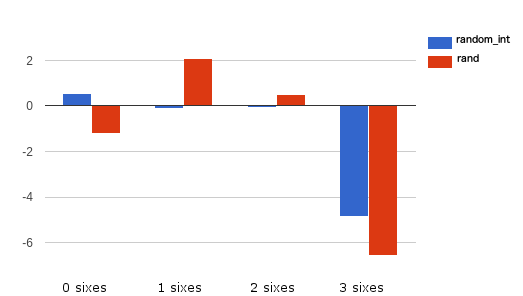# Randomness in PHP - Do You Feel Lucky?

PHP

Share:

#### Free JavaScript Book!Write powerful, clean and maintainable JavaScript.

RRP \$11.95This article analyzes problems related to random number generation used for cryptography purposes. PHP 5 does not provide an easy mechanism for generating cryptographically strong random numbers, while PHP 7 solves this by introducing a couple of CSPRNG functions.

## What Is a CSPRNG?

Quoting Wikipedia, a Cryptographically Secure Pseudorandom Number Generator (CSPRNG) is a pseudo-random number generator (PRNG) with properties that make it suitable for use in cryptography.

A CSPRNG could be mainly useful for:

• Key generation (e.g. generation of complicated keys)
• Creating random passwords for new user accounts
• Encryption systems

A central aspect to keeping a high security level is the high quality of randomness.

## CSPRNG in PHP 7

PHP 7 introduces two new functions that can be used for CSPRNG: `random_bytes` and `random_int`.

The `random_bytes` function returns a `string` and accepts as input an `int` representing the length in bytes to be returned.

Example:

``````\$bytes = random_bytes('10');
var_dump(bin2hex(\$bytes));
//possible ouput: string(20) "7dfab0af960d359388e6"  ``````

The `random_int` function returns an integer number within the given range.
Example:

``````var_dump(random_int(1, 100));
//possible output: 27``````

## Behind the Scenes

The sources of randomness of the above functions are different depending on the environment:

• On Windows, `CryptGenRandom()` will always be used.
• On other platforms, `arc4random_buf()` will be used if it is available (true on BSD derivatives or systems with libbsd).
• Failing the above, a Linux getrandom(2) syscall will be used.
• If all else fails /dev/urandom will be used as the final fallback.
• If none of the above sources are available, then an `Error` will be thrown.

## A Simple Test

A good random number generation system assures the right “quality” of generations. To check this quality, a battery of statistical tests is often performed. Without delving into complex statistical topics, comparing a known behavior with the result of a number generator can help in a quality evaluation.

One easy test is the dice game. Assuming the odds of rolling a six with one die are one in six, if I roll three dice at the same time 100 times, the expected values for 0, 1, 2, and 3 sixes are roughly:

• 0 sixes = 57.9 times
• 1 sixes = 34.7 times
• 2 sixes = 6.9 times
• 3 sixes = 0.5 times

Here is the code to reproduce the dice roll 1.000.000 times:

``````\$times = 1000000;
\$result = [];
for (\$i=0; \$i<\$times; \$i++){
\$dieRoll = array(6 => 0); //initializes just the six counting to zero
\$dieRoll[roll()] += 1; //first die
\$dieRoll[roll()] += 1; //second die
\$dieRoll[roll()] += 1; //third die
\$result[\$dieRoll] += 1; //counts the sixes
}
function roll(){
return random_int(1,6);
}
var_dump(\$result);``````

Testing the code above with PHP7 `random_int` and the simple `rand` function might produce:

Sixes expected `random_int` `rand`
0 579000 579430 578179
1 347000 346927 347620
2 69000 68985 69586
3 5000 4658 4615

To see a better comparison between `rand` and `random_int` we can plot the results in a graph applying a formula to increase the differences between values: `php result - expected result / sqrt(expected)`.

The resulting graph will be:(values close to zero are better)

Even if the three sixes combination doesn’t perform well, and the test is too easy for a real application we can clearly see that `random_int` performs better than `rand`.
Furthermore, the security of an application is increased by the absence of predictable, repeatable behaviors in the random number generator adopted.

By default, PHP 5 does not provide any strong pseudo-random number generators. In reality, there are some options like `openssl_random_pseudo_bytes()`, `mcrypt_create_iv()` or directly use the `/dev/random` or `/dev/urandom` devices with `fread()`. There are also packages like RandomLib or libsodium.

If you want to start using a good random number generator and at the same time be PHP 7-ready you can use the Paragon Initiative Enterprises `random_compat` library. The `random_compat` library allows the use of `random_bytes()` and `random_int()` in your PHP 5.x project.

The library can be installed via Composer:

``composer require paragonie/random_compat``
``````require 'vendor/autoload.php';
\$string = random_bytes(32);
var_dump(bin2hex(\$string));
\$int = random_int(0,255);
var_dump(\$int);
// int(81)``````

The `random_compat` library uses a different preference order compared to the PHP 7 one:

1. `fread()` `/dev/urandom` if available
2. `mcrypt_create_iv(\$bytes, MCRYPT_CREATE_IV)`
3. `COM('CAPICOM.Utilities.1')->GetRandom()`
4. `openssl_random_pseudo_bytes()`

A simple use of the library to generate a password can be:

``````\$passwordChar = '0123456789abcdefghijklmnopqrstuvwxyzABCDEFGHIJKLMNOPQRSTUVWXYZ';
for (\$i = 0; \$i < \$passwordLength; ++\$i) {
}
//possible output: 7rgG8GHu``````

## Conclusion

You should always apply a cryptographically secure pseudo-random number generator, and the `random_compat` lib provides a good implementation for this.

If you want to use a reliable random data source, as you saw in the article, the suggestion is to start as soon as possible with `random_int` and `random_bytes`.

Questions or comments? Leave them below!

Die Hard Test https://en.wikipedia.org/wiki/Diehard_tests
Chi-square test with dice example http://bit.ly/1Mrptf5
Kolmogorov-Smirnov Test https://en.wikipedia.org/wiki/Kolmogorov-Smirnov_test
Spectral Test http://random.mat.sbg.ac.at/tests/theory/spectral/
RaBiGeTe test suite http://cristianopi.altervista.org/RaBiGeTe_MT
Random Number Generation In PHP (2011) http://blog.ircmaxell.com/2011/07/random-number-generation-in-php.html
Testing RNG part 1 and 2 http://ubm.io/1Ot46vL http://ubm.io/1VNzh3N

### Acknowledgements

Many thanks to the following peer reviewers for their help with this article!### Nicola Pietroluongo

Nicola Pietroluongo is a software engineer with many years of experience, open source enthusiast, now creating and contributing to awesome PHP web projects.

#### New books out now!